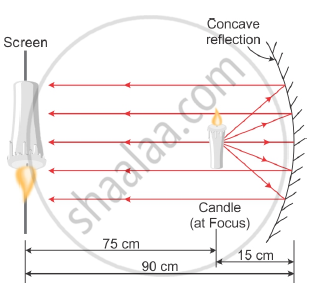Share

# A student wants to project the image of a candle flame on a screen 90 cm in front of a mirror by keeping the flame at a distance of 15 cm from its pole. - CBSE Class 10 - Science

ConceptMirror Formula and Magnification

#### Question

A student wants to project the image of a candle flame on a screen 90 cm in front of a mirror by keeping the flame at a distance of 15 cm from its pole.

(a) Suggest the type of mirror he should use.

(b) Determine the linear magnification in this case.

(c) Find the distance between the object and its image.

(d) Draw ray diagram to show the image formation in this case

#### Solution

(a) Concave mirror

(b) Linear magnification of a concave mirror is given by :-

m="-v"/"u"

=(-(-90))/((-15))

= -6

(c) The distance between the object and image = 90 – 15 = 75 cm.

(d) Ray diagram:-Is there an error in this question or solution?

#### Video TutorialsVIEW ALL 

Solution A student wants to project the image of a candle flame on a screen 90 cm in front of a mirror by keeping the flame at a distance of 15 cm from its pole. Concept: Mirror Formula and Magnification.
S